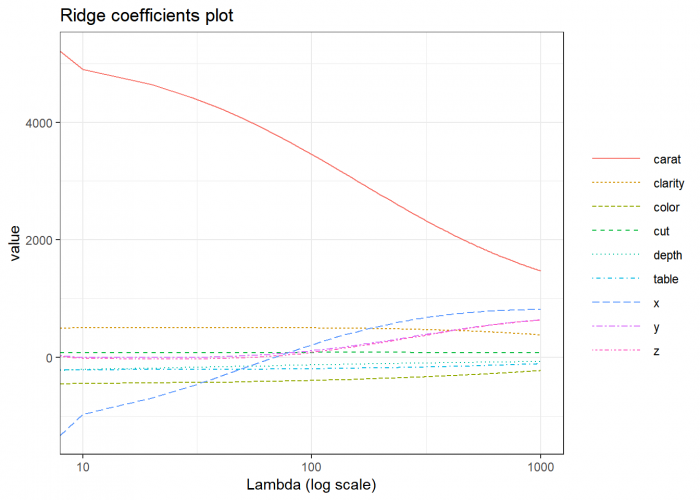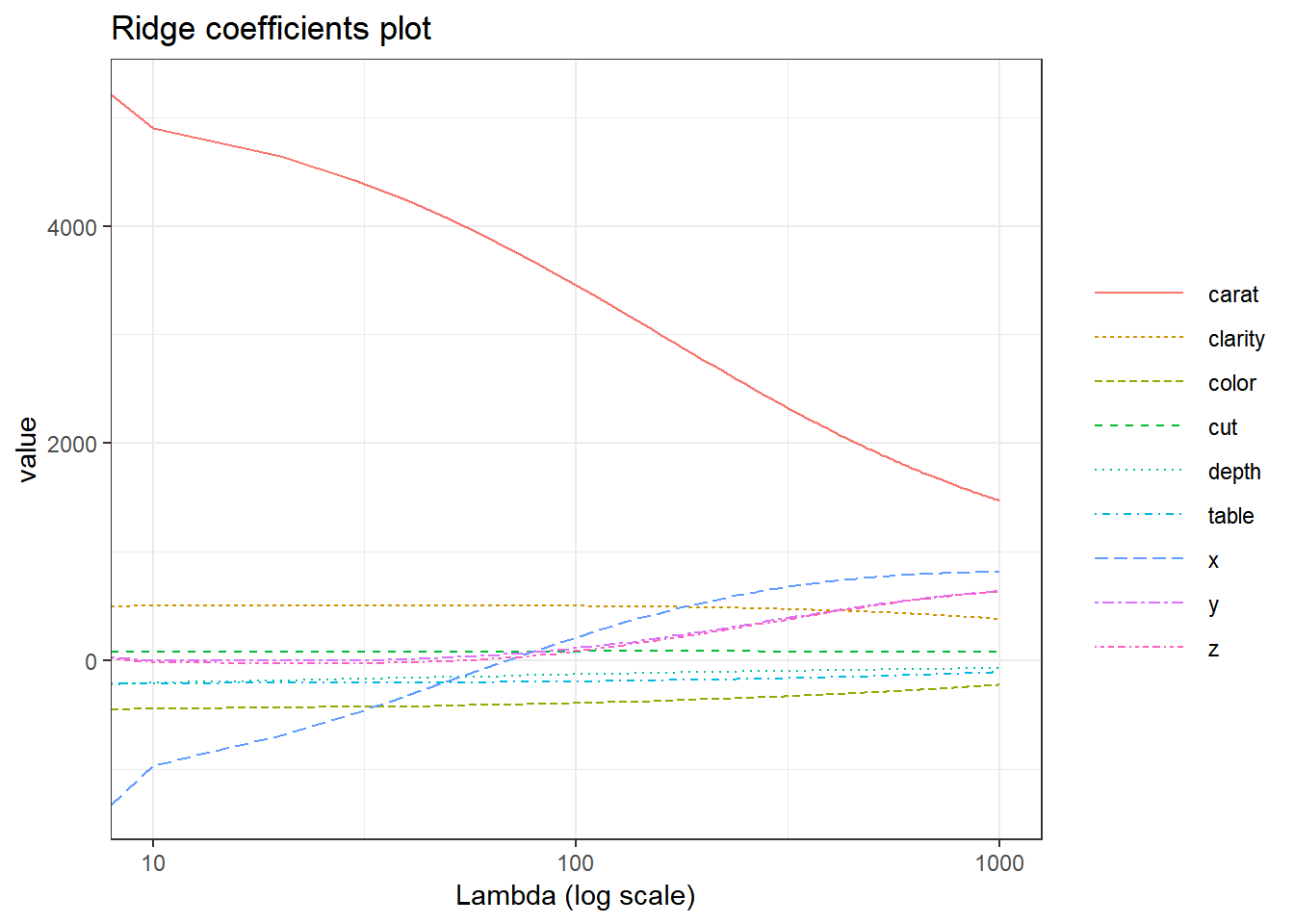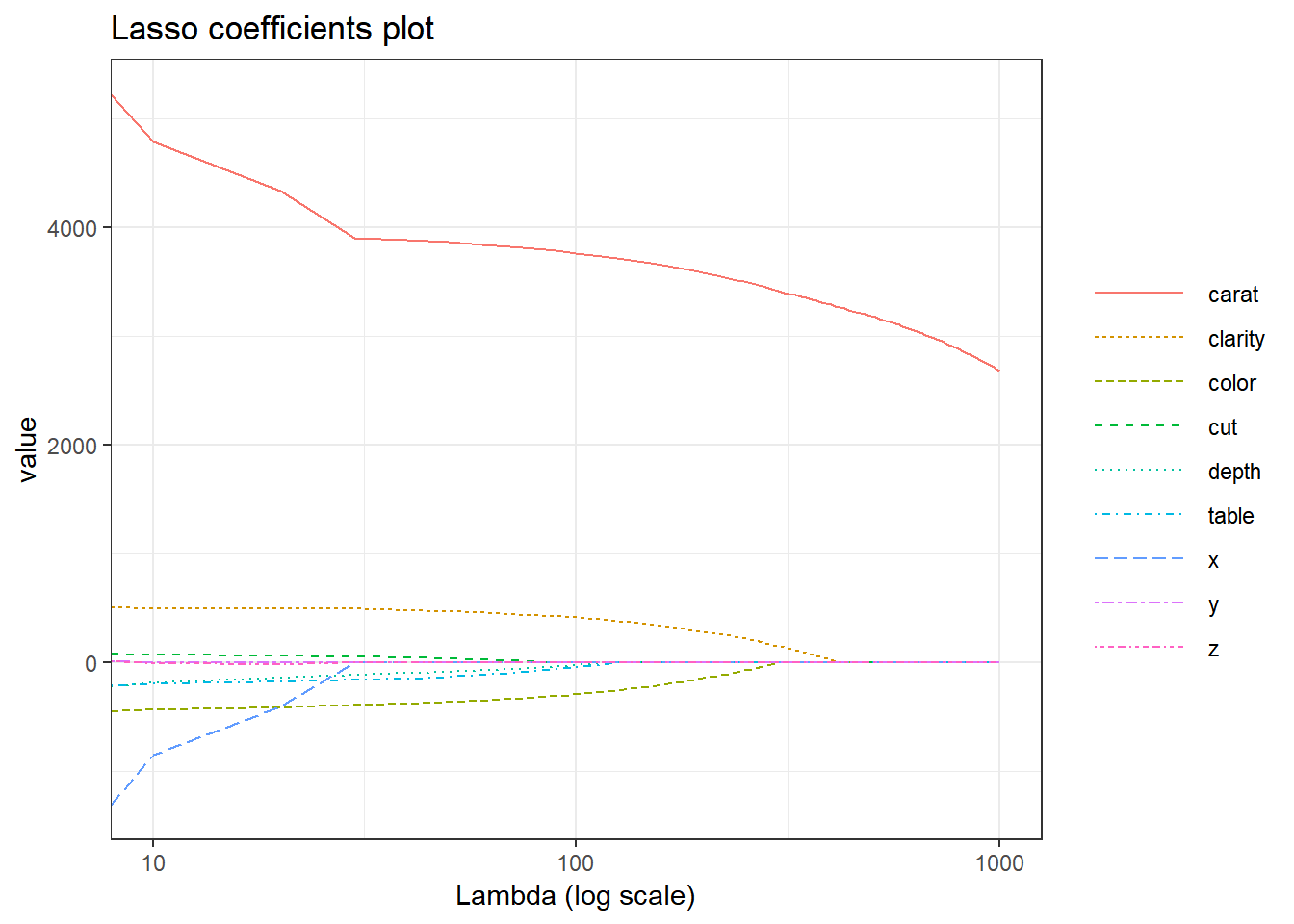# Ridge and Lasso in RA common and very challenging problem in machine learning is overfitting, and it comes in many different appearances. It is one of the major aspects of training the model. Overfitting occurs when the model is capturing too much noise in the training data set which leads to bad predication accuracy when applying the model to new data. One of the ways to avoid overfitting is regularization technique.

In this tutorial, we will examine Ridge regression and Lasso which extend the classical linear regression. Earlier, we have shown how to work with Ridge and Lasso in Python, and this time we will build and train our model using R and the caret package.

Like classical linear regression, Ridge and Lasso also build the linear model, but their fundamental peculiarity is regularization. The goal of these methods is to improve the loss function so that it depends not only on the sum of the squared differences but also on the regression coefficients.

One of the main problems in the construction of such models is the correct selection of the regularization parameter. Comparing to linear regression, Ridge and Lasso models are more resistant to outliers and the spread of data. Overall, their main purpose is to prevent overfitting.

The main difference between Ridge regression and Lasso is how they assign a penalty to the coefficients. We will explore this with our example, so let’s start.We will work with the Diamonds dataset, which you can download from the next website: http://vincentarelbundock.github.io/Rdatasets/datasets.html. It contains the prices and other attributes of almost 54,000 diamonds. We will be predicting the price of diamonds using other attributes and compare the results for Ridge, Lasso, and OLS.

# Upload the dataset
head(diamonds)
##   X carat       cut color clarity depth table price    x    y    z
## 1 1  0.23     Ideal     E     SI2  61.5    55   326 3.95 3.98 2.43
## 2 2  0.21   Premium     E     SI1  59.8    61   326 3.89 3.84 2.31
## 3 3  0.23      Good     E     VS1  56.9    65   327 4.05 4.07 2.31
## 4 4  0.29   Premium     I     VS2  62.4    58   334 4.20 4.23 2.63
## 5 5  0.31      Good     J     SI2  63.3    58   335 4.34 4.35 2.75
## 6 6  0.24 Very Good     J    VVS2  62.8    57   336 3.94 3.96 2.48
# Drop the index column
diamonds<- diamonds[ , -which(names(diamonds)=='X')]
head(diamonds)
##   carat       cut color clarity depth table price    x    y    z
## 1  0.23     Ideal     E     SI2  61.5    55   326 3.95 3.98 2.43
## 2  0.21   Premium     E     SI1  59.8    61   326 3.89 3.84 2.31
## 3  0.23      Good     E     VS1  56.9    65   327 4.05 4.07 2.31
## 4  0.29   Premium     I     VS2  62.4    58   334 4.20 4.23 2.63
## 5  0.31      Good     J     SI2  63.3    58   335 4.34 4.35 2.75
## 6  0.24 Very Good     J    VVS2  62.8    57   336 3.94 3.96 2.48
# Print unique values of text features
print(levels(diamonds$cut)) ##  "Fair" "Good" "Ideal" "Premium" "Very Good" print(levels(diamonds$clarity))
##  "I1"   "IF"   "SI1"  "SI2"  "VS1"  "VS2"  "VVS1" "VVS2"
print(levels(diamonds$color)) ##  "D" "E" "F" "G" "H" "I" "J" As you can see, there is a finite number of variables, so we can change these categorical variables to numerical. diamonds$cut <- as.integer(diamonds$cut) diamonds$color <-as.integer(diamonds$color) diamonds$clarity <- as.integer(diamonds$clarity) head(diamonds) ## carat cut color clarity depth table price x y z ## 1 0.23 3 2 4 61.5 55 326 3.95 3.98 2.43 ## 2 0.21 4 2 3 59.8 61 326 3.89 3.84 2.31 ## 3 0.23 2 2 5 56.9 65 327 4.05 4.07 2.31 ## 4 0.29 4 6 6 62.4 58 334 4.20 4.23 2.63 ## 5 0.31 2 7 4 63.3 58 335 4.34 4.35 2.75 ## 6 0.24 5 7 8 62.8 57 336 3.94 3.96 2.48 Before building the models, let’s first scale data. Scaling will put the ranges of our features from -1 till 1. Thus a feature with a larger scale won’t have a larger impact on the target variable. To scale a feature we need to subtract the mean from every value and divide it by standard deviation. For scaling data and training the models we’ll use caret package. The caret package (short for Classification And REgression Training) is a wrapper around a number of other packages and provides a unified interface for data preparation, models training, and metrics evaluation. # Create features and target matrixes X <- diamonds %>% select(carat, depth, table, x, y, z, clarity, cut, color) y <- diamonds$price

# Scale data
preprocessParams<-preProcess(X, method = c("center", "scale"))
X <- predict(preprocessParams, X)

Now, we can basically build Lasso and Ridge. We’ll split the data into a train and a test dataset but for now we won’t set the regularization parameter lambda. It is set to 1.

The “glmnet” method in caret has an alpha argument that determines what type of model is fit. If alpha = 0 then a ridge regression model is fit, and if alpha = 1 then a lasso model is fit. Here we’ll use caret as a wrapper for glment.

# Spliting training set into two parts based on outcome: 75% and 25%
index <- createDataPartition(y, p=0.75, list=FALSE)
X_train <- X[ index, ]
X_test <- X[-index, ]
y_train <- y[index]
y_test<-y[-index]
# Create and fit Lasso and Ridge objects
lasso<-train(y= y_train,
x = X_train,
method = 'glmnet',
tuneGrid = expand.grid(alpha = 1, lambda = 1)

)

ridge<-train(y = y_train,
x = X_train,
method = 'glmnet',
tuneGrid = expand.grid(alpha = 0, lambda = 1)

)

# Make the predictions
predictions_lasso <- lasso %>% predict(X_test)
predictions_ridge <- ridge %>% predict(X_test)

# Print R squared scores
data.frame(
Ridge_R2 = R2(predictions_ridge, y_test),
Lasso_R2 = R2(predictions_lasso, y_test)
)
##    Ridge_R2  Lasso_R2
## 1 0.8584854 0.8813328
# Print RMSE
data.frame(
Ridge_RMSE = RMSE(predictions_ridge, y_test) ,
Lasso_RMSE = RMSE(predictions_lasso, y_test)
)
##   Ridge_RMSE Lasso_RMSE
## 1   1505.114   1371.852
# Print coeficients
data.frame(
as.data.frame.matrix(coef(lasso$finalModel, lasso$bestTune$lambda)), as.data.frame.matrix(coef(ridge$finalModel, ridge$bestTune$lambda))
) %>%
rename(Lasso_coef = X1, Ridge_coef = X1.1)
##              Lasso_coef Ridge_coef
## (Intercept)  3934.27842 3934.23903
## carat        5109.77597 2194.54696
## depth        -210.68494  -92.16124
## table        -207.38199 -156.89671
## x           -1178.40159  709.68312
## y               0.00000  430.43936
## z               0.00000  423.90948
## clarity       499.77400  465.56366
## cut            74.61968   83.61722
## color        -448.67545 -317.53366

These two models give very similar results. Now let’s choose the regularization parameter with the help of tuneGrid. The models with the highest R-squared score will give us the best parameters.

parameters <- c(seq(0.1, 2, by =0.1) ,  seq(2, 5, 0.5) , seq(5, 25, 1))

lasso<-train(y = y_train,
x = X_train,
method = 'glmnet',
tuneGrid = expand.grid(alpha = 1, lambda = parameters) ,
metric =  "Rsquared"
)

ridge<-train(y = y_train,
x = X_train,
method = 'glmnet',
tuneGrid = expand.grid(alpha = 0, lambda = parameters),
metric =  "Rsquared"

)
linear<-train(y = y_train,
x = X_train,
method = 'lm',
metric =  "Rsquared"
)

print(paste0('Lasso best parameters: ' , lasso$finalModel$lambdaOpt))
##  "Lasso best parameters: 2.5"
print(paste0('Ridge best parameters: ' , ridge$finalModel$lambdaOpt))
##  "Ridge best parameters: 25"
predictions_lasso <- lasso %>% predict(X_test)
predictions_ridge <- ridge %>% predict(X_test)
predictions_lin <- linear %>% predict(X_test)

data.frame(
Ridge_R2 = R2(predictions_ridge, y_test),
Lasso_R2 = R2(predictions_lasso, y_test),
Linear_R2 = R2(predictions_lin, y_test)
)
##    Ridge_R2  Lasso_R2 Linear_R2
## 1 0.8584854 0.8813317 0.8813157
data.frame(
Ridge_RMSE = RMSE(predictions_ridge, y_test) ,
Lasso_RMSE = RMSE(predictions_lasso, y_test) ,
Linear_RMSE = RMSE(predictions_ridge, y_test)
)
##   Ridge_RMSE Lasso_RMSE Linear_RMSE
## 1   1505.114   1371.852    1505.114
print('Best estimator coefficients')
##  "Best estimator coefficients"
data.frame(
ridge = as.data.frame.matrix(coef(ridge$finalModel, ridge$finalModel$lambdaOpt)), lasso = as.data.frame.matrix(coef(lasso$finalModel, lasso$finalModel$lambdaOpt)),
linear = (linear$finalModel$coefficients)
) %>%   rename(lasso = X1, ridge = X1.1)
##                  lasso       ridge      linear
## (Intercept) 3934.23903  3934.27808  3934.27526
## carat       2194.54696  5103.53988  5238.57024
## depth        -92.16124  -210.18689  -222.97958
## table       -156.89671  -207.17217  -210.64667
## x            709.68312 -1172.30125 -1348.94469
## y            430.43936     0.00000    23.65170
## z            423.90948     0.00000    22.01107
## clarity      465.56366   499.73923   500.13593
## cut           83.61722    74.53004    75.81861
## color       -317.53366  -448.40340  -453.92366

Our score raised a little, but with these values of lambda, there is not much difference. Let’s build coefficient plots to see how the value of lambda influences the coefficients of both models.

We will use glmnet function to train the models and then we’ll use plot() function that produces a coefficient profile plot of the coefficient paths for a fitted “glmnet” object.

Xvar variable of plot() defines what is on the X-axis, and there are 3 possible values it can take: “norm”, “lambda”, or “dev”, where “norm” is for L1-norm of the coefficients, “lambda” for the log-lambda sequence, and “dev” is the percent deviance explained. We’ll set it to lambda.

To train glment, we need to convert our X_train object to a matrix.

# Set lambda coefficients
paramLasso <- seq(0, 1000, 10)
paramRidge <- seq(0, 1000, 10)

# Convert X_train to matrix for using it with glmnet function
X_train_m <- as.matrix(X_train)

# Build Ridge and Lasso for 200 values of lambda
rridge <- glmnet(
x = X_train_m,
y = y_train,
alpha = 0, #Ridge
lambda = paramRidge

)

llaso <- glmnet(
x = X_train_m,
y = y_train,
alpha = 1, #Lasso
lambda = paramLasso

)
## Warning: Transformation introduced infinite values in continuous x-axis## Warning: Transformation introduced infinite values in continuous x-axisAs a result, you can see that when we raise the lambda in the Ridge regression, the magnitude of the coefficients decreases, but never attains zero. The same scenario in Lasso influences less on the large coefficients, but the small coefficients are reduced to zeroes.

We can conclude from the plot that the “carat”" feature has the most impact on the target variable. Other important features are “clarity” and “color” while features like “z” and “cut” have barely any impact.

Therefore Lasso can also be used to determine which features are important to us and have strong predictive power. The Ridge regression gives uniform penalties to all the features and in such way reduces the model complexity and prevents multicollinearity.